﻿ Bisecting an arbitrary triangular cake# Bisecting an arbitrary triangular cake

Earlier this week we saw how to bisect an equilateral triangular cake. Here's the original posting: Bisecting a triangular cake.

What happens if the bakery poorly eyeballs the dimensions it comes out a bit more scalene?Shortly after publishing my article, I was contacted by Scott Carr, a talented graduate student from Rice University. Scott extended my simple equilateral triangle cake puzzle and solved it for a generic triangle. He sent me a link to his work, and then graciously offered to write it up for me to publish on this blog. Here, in his own words, is his work. Thanks for reaching out.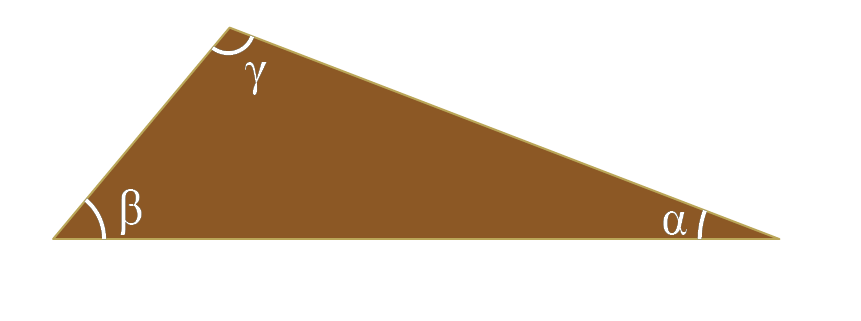The game is the same as before but the players are a bit different. We still would like to cut the cake into two equal pieces using a single, straight cut. We follow a very similar path to before but need to do some careful labeling of sides and angles before diving in. We arrange our cake so that the shortest side is called a, medium side b, and longest side c. Being good geometers (despite the sweet tooth) we dutifully label all the angles: α opposite a, β opposite b, and γ opposite c. Hope you're hungry cause it is time to get mathy!
 We begin with an arbitrary cut through the two sides of the triangle. But which two? On a hunch we cut the two longest sides. Making a cut opposite the smallest angle seems like a good place to start. This cuts gives a funky quadrilateral and a new triangle. We label the sides of the triangle b' on the b edge, c' on the c edge, and L along the cut. For this cut to be an area bisecter, then the area of this new triangle must be half of the area of the whole cake.We know the area of a triangle is half its base multiplied by its height. Applying our trigonomitry skillz, we find the area for the whole cake as well as the triangular slice.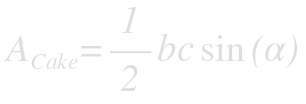Because we are excellent bisecters, we know that the area of the slice will be equal to half of the overall cake. Taking the ratio of the two provides a useful relation between b' and c' in terms of known cake edges b and c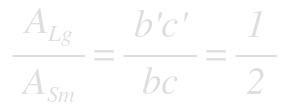The law of cosines provides us a nice expression for the length of the cut(L), in terms of b' and c':Using the ratio of the areas to find an expression for b', we can write L in terms of a single variable, c':To minimize the cut length for a certain angle α, take the derviative of L with respect to c':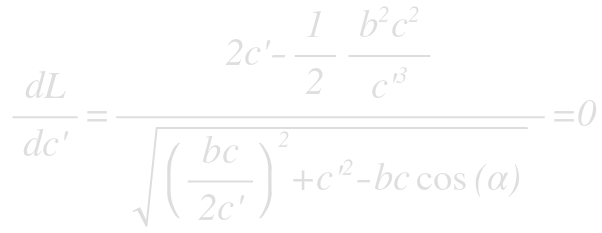Fortunately, only the numerator must be zero. Solving the numerator for c' yields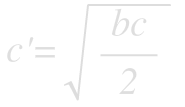which, using the relationship from the area ratios gives.### Result

What we find in the end is that the shortest cut of an arbitrary triangle comes when you cut off an isosceles triangle of equal area. This is a natural generalization of the result from the equilateral cake. In that case, we cut an isosceles triangle that happened to have the third side equal to the other two, making it equilateral.

### Cut Length

Using these equations we can determine the length of the smallest cut by substitution to find:This is super pretty! Checking in with the equilateral triangle (α=60°, b=c=1) we recover the correct value of 1/√2. However, it would be nice to express the length solely in terms of the cake dimensions. This is possible using our good friend the law of cosines to determine cos(α) in terms of a, b, and c. The result is the rather find looking expression:## That's it folks

 Thanks for indulging me in a bit of mathematical fun. Next time you and a buddy order a triangle cake that comes out quite scalene, you now can split it evenly. All this cake talk has made me hungry...

You can find a complete list of all the articles here.Click here to receive email alerts on new articles.